# Tree Implementations Chapter 25 Chapter Contents The Nodes

• Slides: 24
Download presentation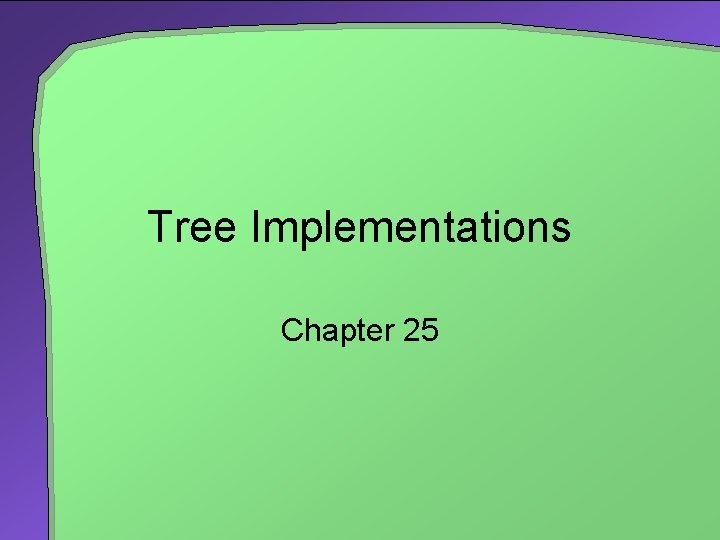Tree Implementations Chapter 25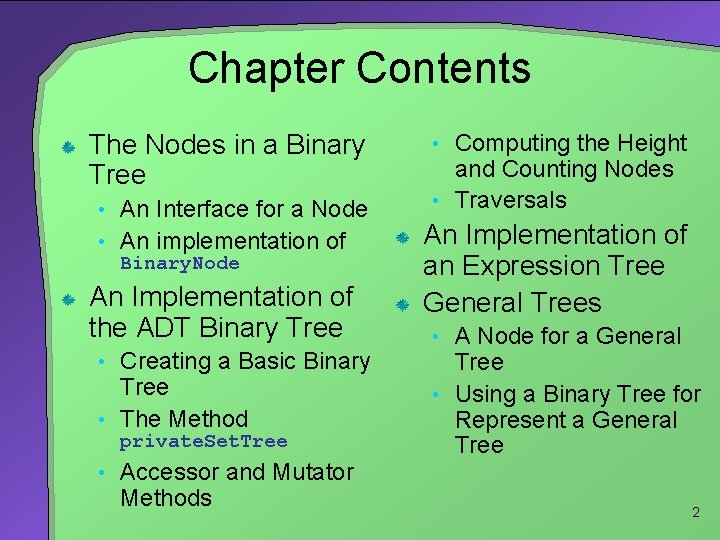Chapter Contents The Nodes in a Binary Tree • An Interface for a Node • An implementation of Binary. Node An Implementation of the ADT Binary Tree • Creating a Basic Binary Tree • The Method private. Set. Tree • Accessor and Mutator Methods • Computing the Height and Counting Nodes • Traversals An Implementation of an Expression Tree General Trees • A Node for a General Tree • Using a Binary Tree for Represent a General Tree 2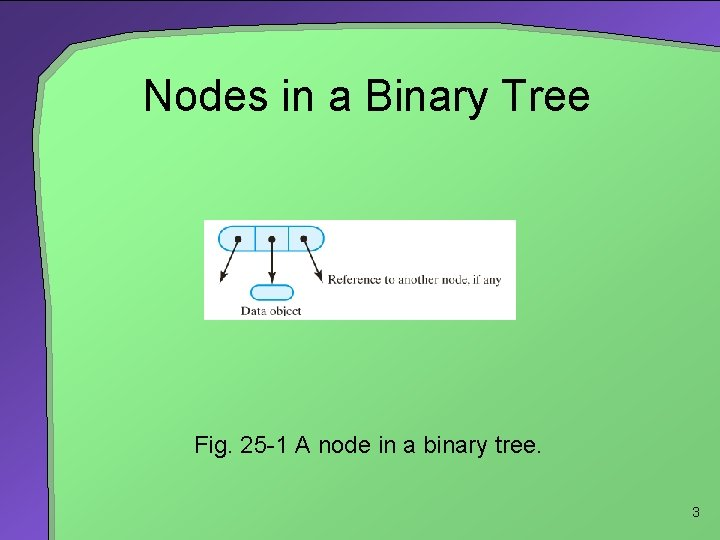Nodes in a Binary Tree Fig. 25 -1 A node in a binary tree. 3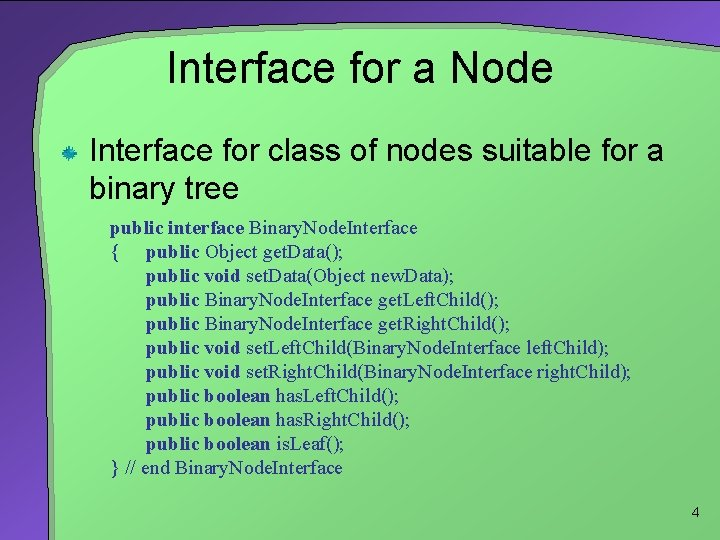Interface for a Node Interface for class of nodes suitable for a binary tree public interface Binary. Node. Interface { public Object get. Data(); public void set. Data(Object new. Data); public Binary. Node. Interface get. Left. Child(); public Binary. Node. Interface get. Right. Child(); public void set. Left. Child(Binary. Node. Interface left. Child); public void set. Right. Child(Binary. Node. Interface right. Child); public boolean has. Left. Child(); public boolean has. Right. Child(); public boolean is. Leaf(); } // end Binary. Node. Interface 4Implementation of Binary. Node Usually the class that represents a node in a tree is a detail hidden from the client It is placed within a package • Makes it available • Available to all classes involved in implementation of a tree 5An Implementation of the ADT Binary Tree Recall interface for class of binary trees from previous chapter In that interface • Tree. Interface specifies basic operations common to all trees • Tree. Interator. Interface specifies operations for tree traversal Note full implementation of Binary. Tree, section 25. 4 in text. 6The Method private. Set. Tree Problem: previous implementation of private. Set. Tree not sufficient Given: tree. A. set. Tree (a, tree. B, tree. C); • Now tree. A shares nodes with tree. B, tree. C • Changes to tree. B also affect tree. A (Fig. 25 -2) fig 25 -2 here 7The Method private. Set. Tree Possible solution • Have privat. Set. Tree copy the nodes in Tree. B and Tree. C Now tree. A is separate and distinct from tree. B and tree. C • Changes to either tree. B or tree. C do NOT affect tree. A Note that copying nodes is expensive • Other solutions are considered 8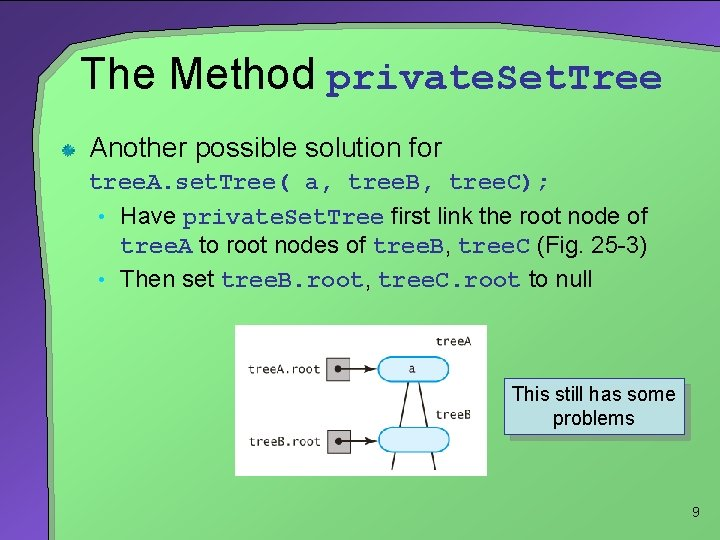The Method private. Set. Tree Another possible solution for tree. A. set. Tree( a, tree. B, tree. C); • Have private. Set. Tree first link the root node of tree. A to root nodes of tree. B, tree. C (Fig. 25 -3) • Then set tree. B. root, tree. C. root to null fig 25 -3 here This still has some problems 9The Method private. Set. Tree Real solution should do the following: Create root node r for containing the data If left subtree exists, not empty 1. 2. • Attach root node to r as left child If right subtree exists, not empty, distinct from left subtree 3. • • Attach root node r as right child If right, left subtrees are the same, attach copy of right subtree to r instead If left (right) subtree exists, different from the invoking tree object 4. • Set its data field root to null 10Accessor, Mutator Methods implemented • get. Root. Data() • is. Empty() • clear() • set. Root. Data (Object root. Data) • set. Root. Node (Binary. Node root. Node) • get. Root. Node() 11Computing Height, Counting Nodes Within Binary. Tree • get. Height() • get. Number. Of. Nodes() Within Binary. Node • get. Height() • get. Height(Binary. Node node) • get. Number. Of. Nodes() 12Traversals Make recursive method private • Call from public method with no parameters public void inorder. Traverse() { inorder. Traverse(root); } private void inorder. Traverse(Binary. Node node) { if (node != null) { inorder. Traverse((Binary. Node)node. get. Left. Child()); System. out. println(node. get. Data()); inorder. Traverse((Binary. Node)node. get. Right. Child()); } // end if } // end inorder. Traverse 13Traversals Fig. 25 -4 A binary tree. 14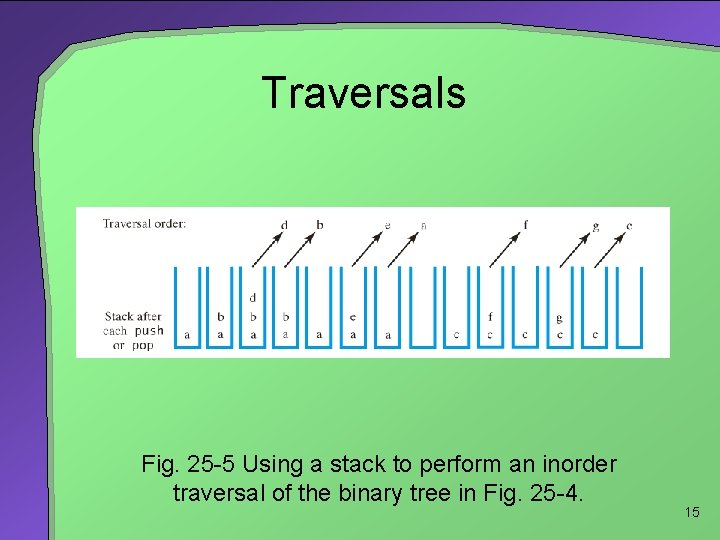Traversals Fig. 25 -5 Using a stack to perform an inorder traversal of the binary tree in Fig. 25 -4. 15Traversals Fig. 25 -6 Using a stack to traverse the binary tree in Fig. 25 -4 in (a) preorder 16Traversals Fig. 25 -6 Using a stack to traverse the binary tree in Fig. 25 -4 in (b) postorder. 17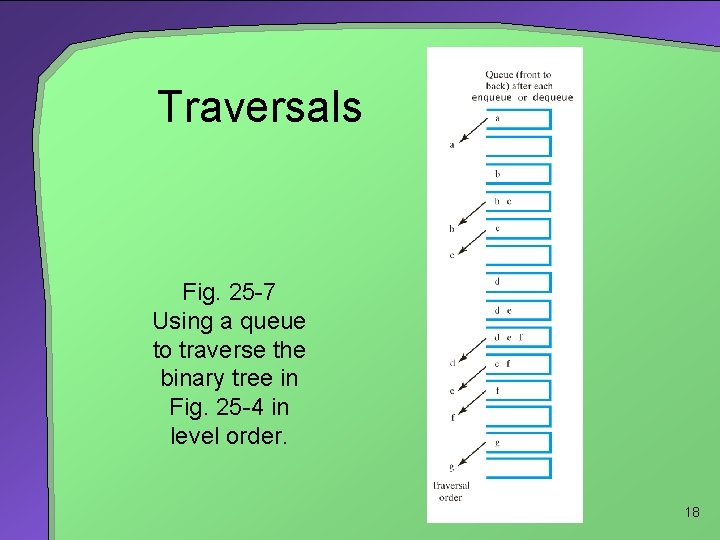Traversals Fig. 25 -7 Using a queue to traverse the binary tree in Fig. 25 -4 in level order. 18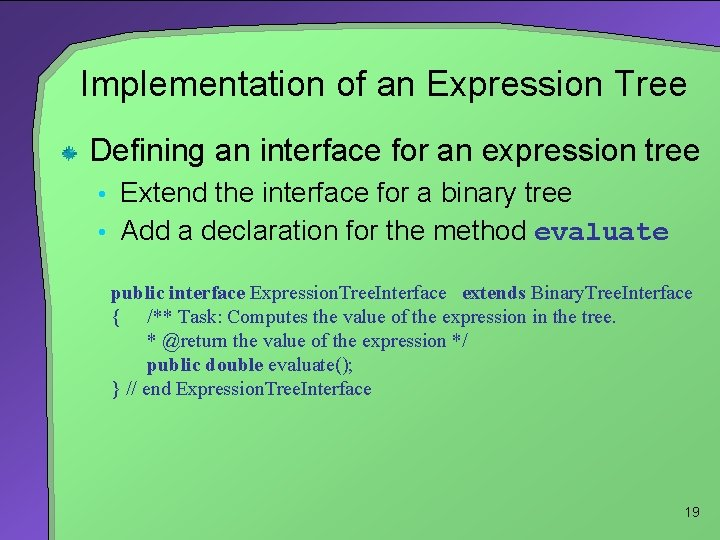Implementation of an Expression Tree Defining an interface for an expression tree • Extend the interface for a binary tree • Add a declaration for the method evaluate public interface Expression. Tree. Interface extends Binary. Tree. Interface { /** Task: Computes the value of the expression in the tree. * @return the value of the expression */ public double evaluate(); } // end Expression. Tree. Interface 19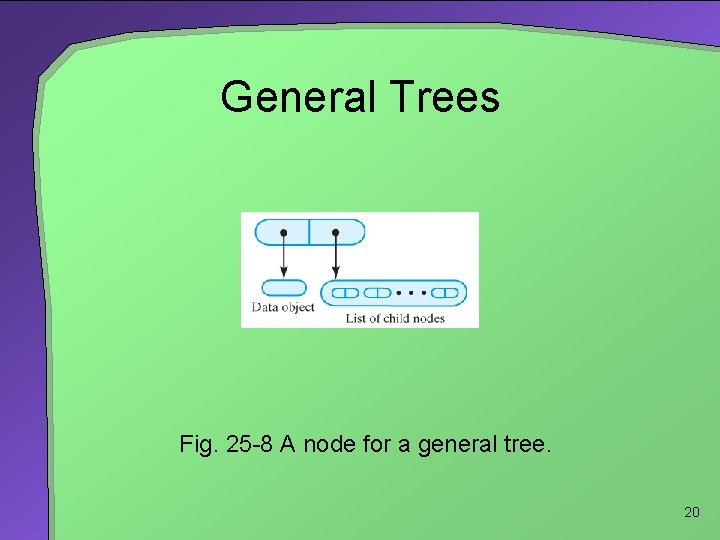General Trees Fig. 25 -8 A node for a general tree. 20Using a Binary Tree to Represent a General Tree Fig. 25 -9 (a) A general tree; 21Using a Binary Tree to Represent a General Tree Fig. 25 -9 (b) an equivalent binary tree; 22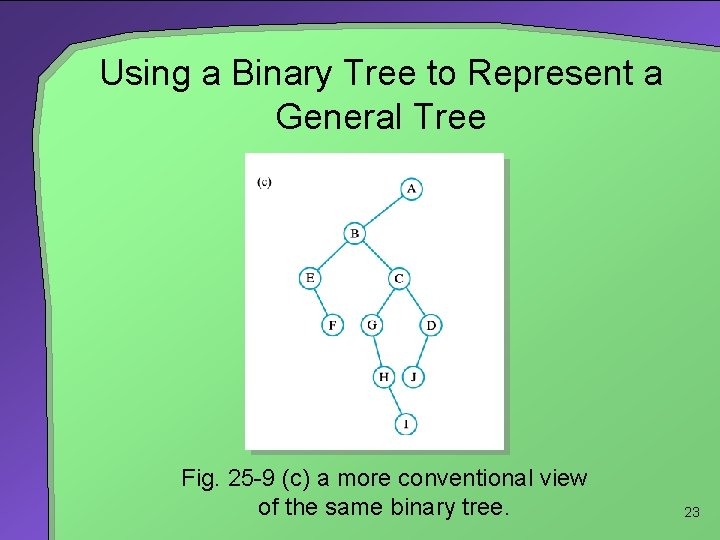Using a Binary Tree to Represent a General Tree Fig. 25 -9 (c) a more conventional view of the same binary tree. 23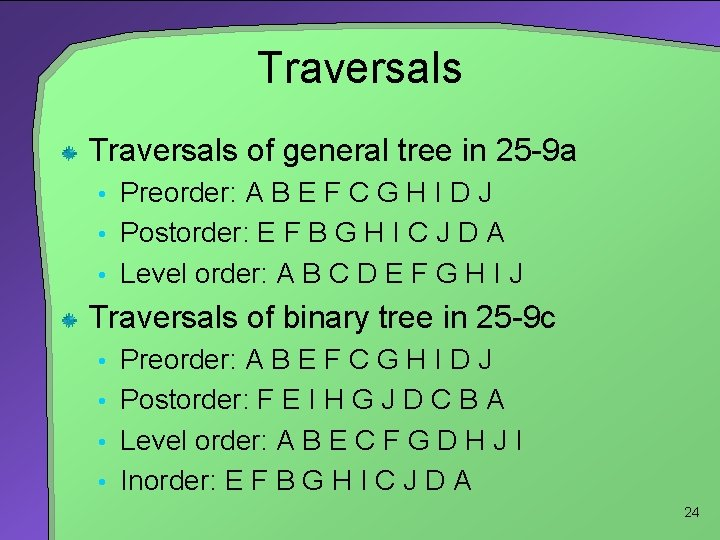Traversals of general tree in 25 -9 a • Preorder: A B E F C G H I D J • Postorder: E F B G H I C J D A • Level order: A B C D E F G H I J Traversals of binary tree in 25 -9 c • Preorder: A B E F C G H I D J • Postorder: F E I H G J D C B A • Level order: A B E C F G D H J I • Inorder: E F B G H I C J D A 24